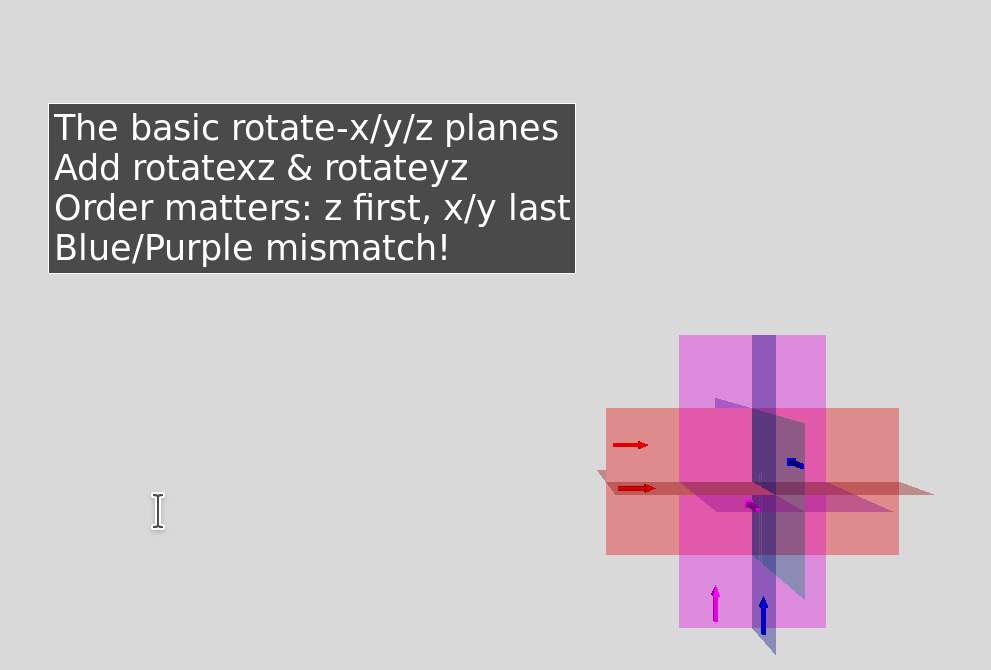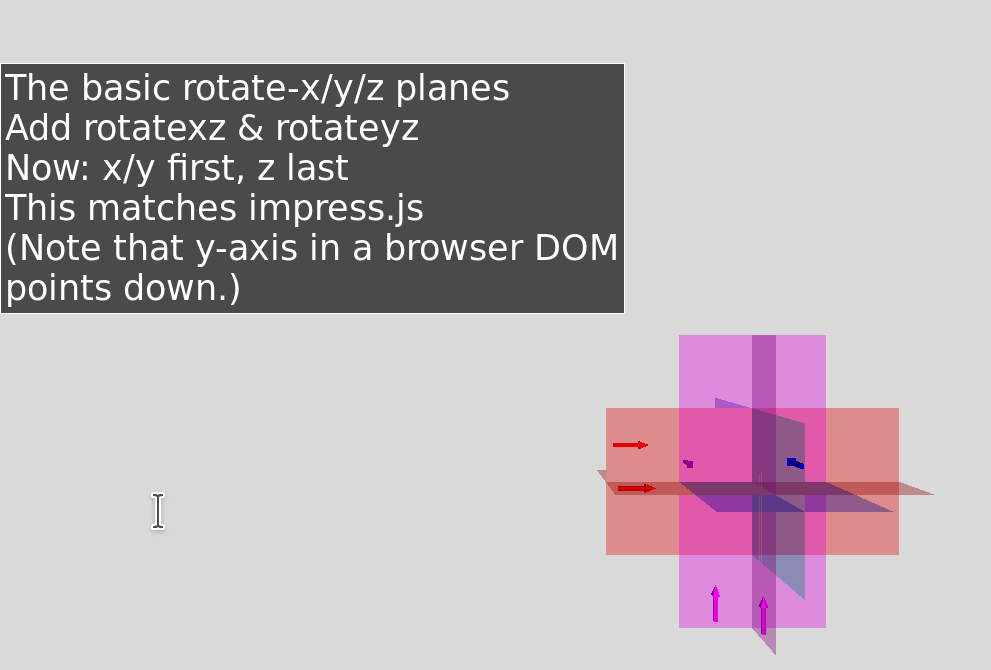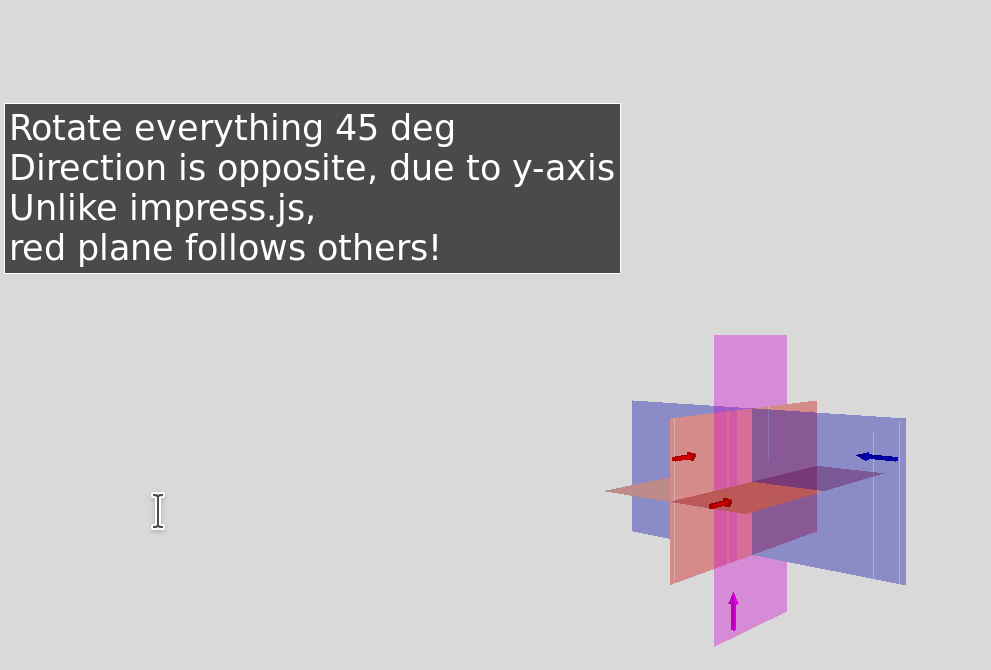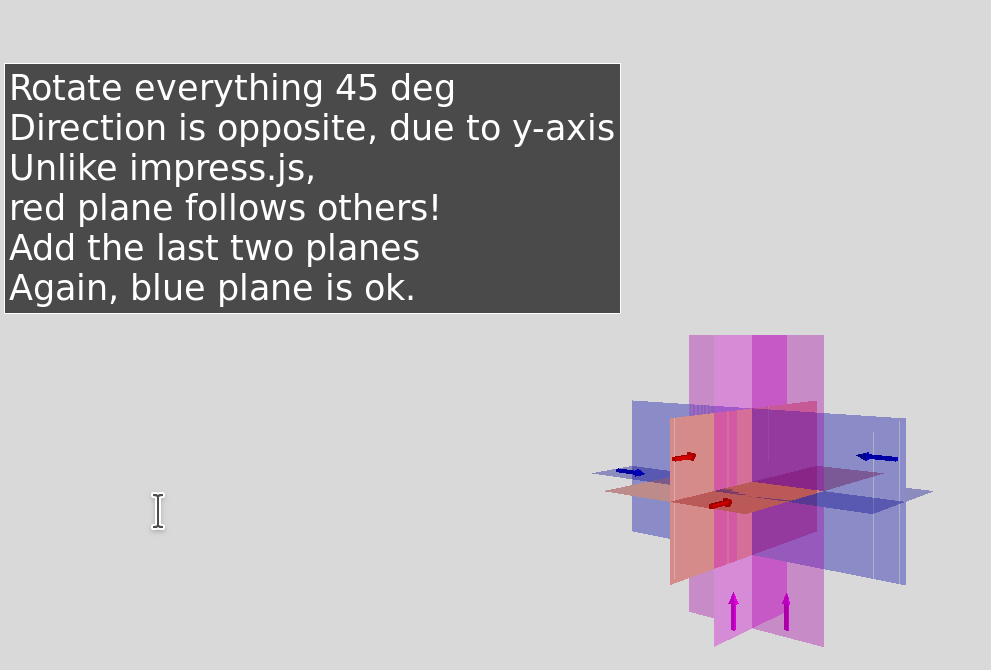# 3D study

### Various 3D geometries in impress.js

Note: Click here to skip steps without graphics:
Next...

90 degree rotations around origin:

• XY-plane (all coordinates 0)
• RotateX=90
• RotateY=90
• RotateZ=90
• RotateX, RotateZ =90
• RotateY, RotateZ =90

Note: dotted lines are the same (left, top) edges.
Looks like the blue ones are not the same way!

Next...

90 degree rotations around origin:

• XY-plane (all coordinates 0)
• RotateX=90
• RotateY=90
• RotateZ=90
• RotateX=90, RotateZ=-90
• RotateY, RotateZ =90

Note: dotted lines are the same (left, top) edge.
Looks like the blue ones are not the same way!
Now that's better. (RotateZ=-90)

Except it's still not perfect. The red ones are
rotated counter-clockwise (wired vs filled),
while the blue and purple pairs are rotated clockwise.

Next...

90 degree rotations around origin:

• XY-plane (all coordinates 0)
• RotateX=90
• RotateY=90
• RotateZ=90
• RotateX=90, RotateZ=-90, RotateY=180
• RotateY=-90, RotateZ=90

This is what we'd have to do to have all of them
rotate the same way.

We will not use this for now.

Next...

Now rotate the filled planes 45 degrees around y-axis:

• XY-plane
• RotateX=90 RotateY=45
• RotateY=90 +45 = 135
• RotateZ=90 RotateY=45
• RotateX,Z =90
• RotateY,Z =90

So let's go back to the simple geometry,
where everything was rotated +90, and rotate +45
along y-axis

Wait, what's wrong with the red (RotateX=90) plane?

Next...

Now rotate the filled planes 45 degrees around y-axis:

• XY-plane
• RotateX=90 RotateZ=-45
• RotateY=90 +45 = 135
• RotateZ=90 RotateY=45
• RotateX,Z =90
• RotateY,Z=90

Setting RotateZ=-45 fixes the red plane. (Why?)
Ok, let's bring back the non-filled planes...

Next...

Now rotate all planes 45 degrees around y-axis:

• XY-plane RotateY=45
• RotateX=90 RotateZ=-45
• RotateY=90 +45 = 135
• RotateZ=90 RotateY=45
• RotateX,Z=90 RotateY=45
• RotateZ=90 RotateY=90+45=135

Setting RotateZ=-45 fixes the red plane. (Why?)
Ok, let's bring back the non-filled planes and add RotateY=45
Now the blue one is off!

Next...

Now rotate all planes 45 degrees around y-axis:

• XY-plane RotateY=45
• RotateX=90 RotateZ=-45
• RotateY=90 +45 = 135
• RotateZ=90 RotateY=45
• RotateX=90, RotateZ=90-45= -45
• RotateZ=90 RotateY=90+45=135

Wait, what's wrong with the red (RotateX=90) plane?
Setting RotateZ=-45 fixes the red plane. (Why?)
Ok, let's bring back the non-filled planes and add RotateY=45
Now the blue one is off!
Setting an additional -45 on z-axis puts it right.

Next...

Now rotate all planes 45 degrees around y-axis:

• XY-plane RotateY=45
• RotateX=90 RotateZ=-45
• RotateY=90 +45 = 135
• RotateZ=90 RotateY=45
• RotateX=90, RotateZ=90-45= -45
• RotateZ=90 RotateY=90+45=135

So what is the conclusion?
RotateX=90 causes something odd to happen, on those we
must add RotateZ= -α instead of RotateY=α

Next...

# But wait, there's more

### Until now, we still left some combinations out.

Next...

90 degree rotations around origin:

• XY-plane (all coordinates 0)
• RotateX=90
• RotateY=90
• RotateZ=90
• RotateX, RotateZ =90
• RotateX, RotateZ =90
• RotateY, RotateZ =90
• RotateX, Y, Z =90

Newly added rectangles are shifted +30 on the x-axis
because they're overlapping with already existing rectangles.

Observation: RotateX,Z (green) is the same rectangle as
RotateY,Z (purple). RotateX,Y,Z (black) is the same as
RotateY (blue-fill), except it's in opposite direction.

Next...

I'm not good enough with math to claim the above behavior is a bug.

But I'm certainly used to math being more consistent and simple than this!

Let's compare to a reference implementation used by scientists.

Same transformations in Visual Python 3D framework.

Next...Next...Next...Next...Next...Next...

Conclusion: When data-rotate-x is used, it distorts relationship between data-rotate-y and -z.

Is it a bug? If yes, is it in impress.js or the browser DOM? Latter would be unlikely...

Continue here to explore the same problem in plain css.

Bonus: Exploring what rotate3d() does. (Didn't make me much wiser.)